## Types of Clocks, How to Tell Time, Conversion

### Clocks

Digital clocks and watches have digits like 0,1,2,3 ...59

Digital Clocks show us the time using numbers, like this:
HOURS : MINUTES
We say that the time is 2:29, 10:26 or 1:10 etc.

A.M. is from 12:01 at night until 11:59 in the morning. P.M. is from 12:00 in the day until 12:00 at night.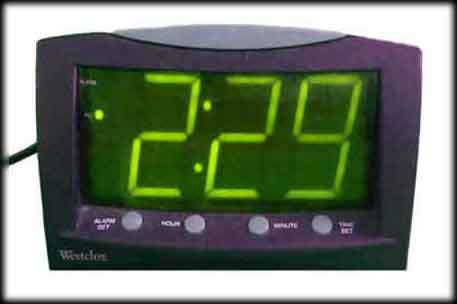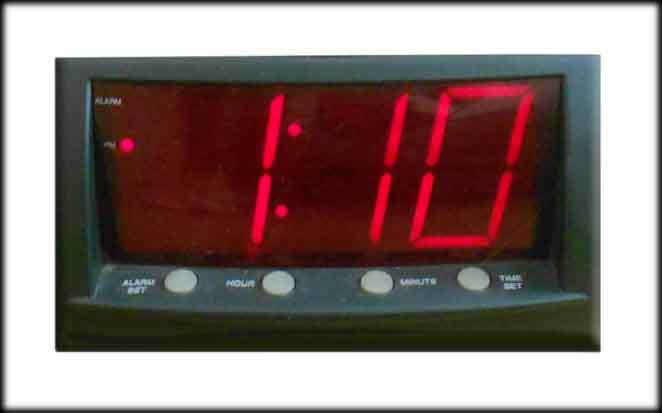Analog clocks and watches have hands. The small hand indicates the hour, and the big hand indicates the minute. As the big hand moves around the 60 minutes of the clock, the small hand slowly moves from one hour to the next. A clock face or dial may also include a second hand. It is very thin and moves very fast. Every time it moves, a second has gone by.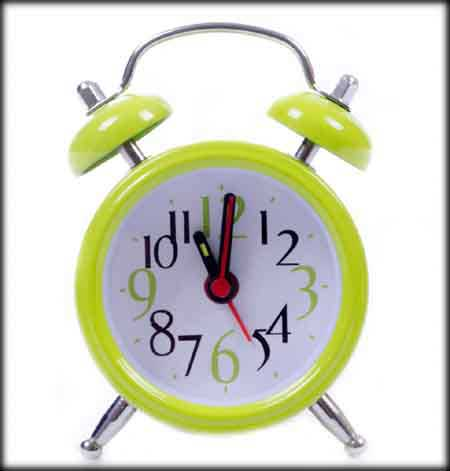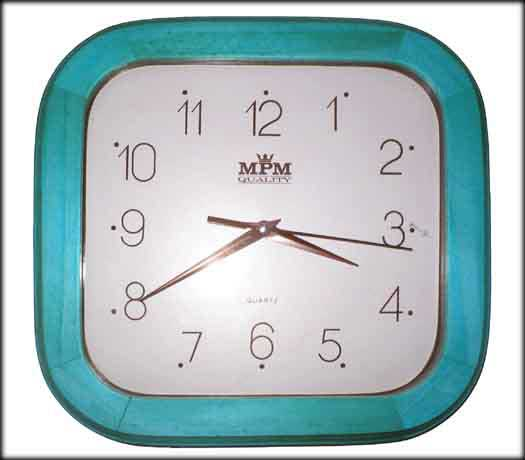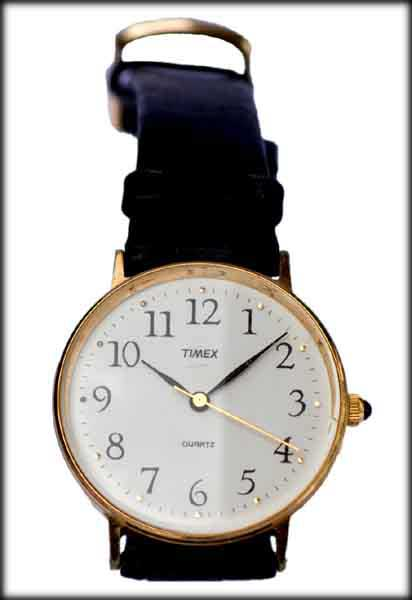The lines or dots between the numbers on the clock represent the 60 minutes that make up one hour.

Every hand on a clock travels around a circle in the same direction. We call this direction "clockwise." It goes in the order of the numbers, like the clock was counting up from 1 to 12. The hands on the clock always travel this direction when they are working properly.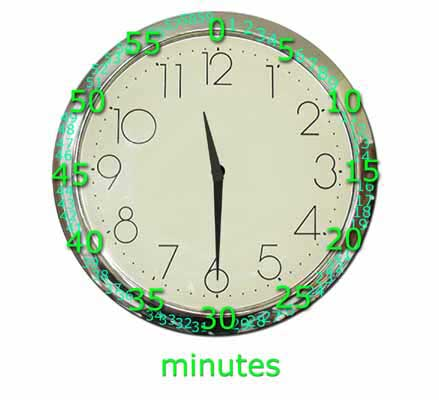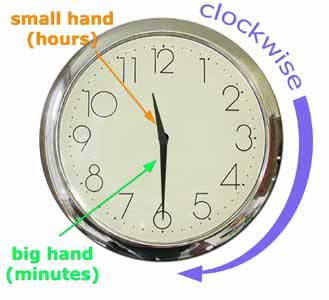## How to Tell Time- the Easy Method

Tell the time by first looking at the small hand of the clock and determine the hour. If the hours hand is pointed in between two numbers, use the lower number. Then look at the big hand of the clock and determine the minute. In your mind, extend the minute hand all the way to the edge, and read/count how many minutes it has passed.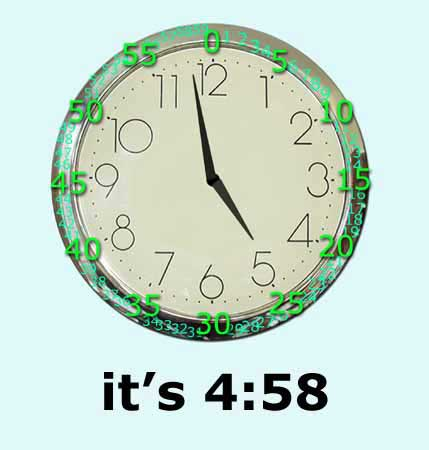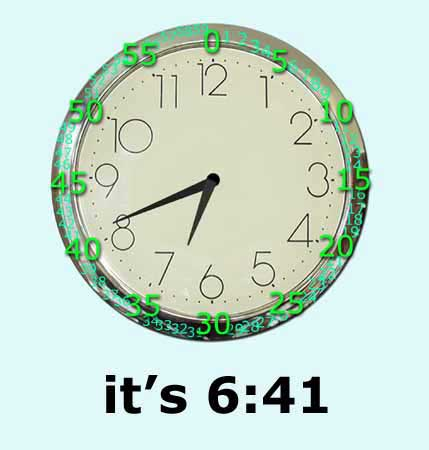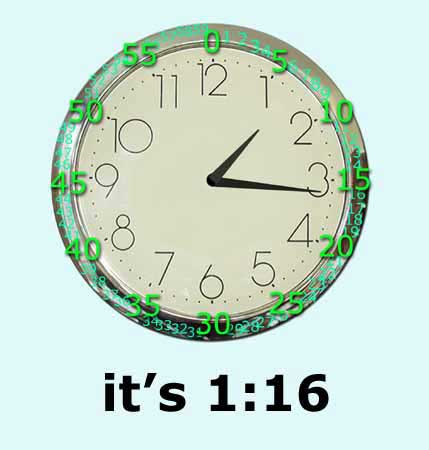## How to Tell Time- the Not So Easy Method

### When minute hand is pointing to 12, 3, 6 or 9

#### example 1

When the minute hand is pointing to 12 and the hours hand is pointing directly at a big number, it's exactly that-o'clock.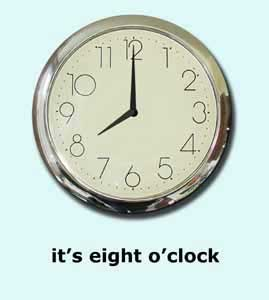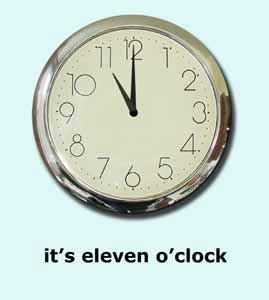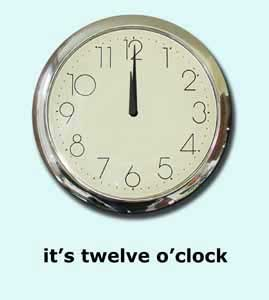#### example 2

When the minute hand is pointing to 3, we say that the time is "a quarter after/past _____."
The hours hand will be pointed in between two numbers. The hour of the day is the lower number.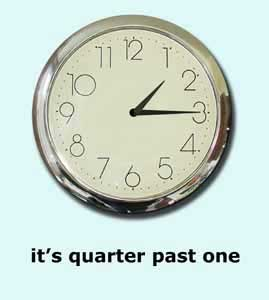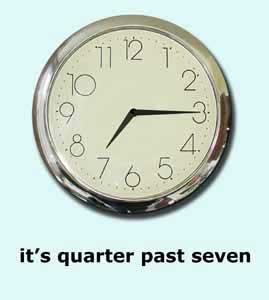#### example 3

When the minute hand is pointing to 6, we say that the time is "half past _____."
The hours hand will be pointed in between two numbers. The hour of the day is the lower number.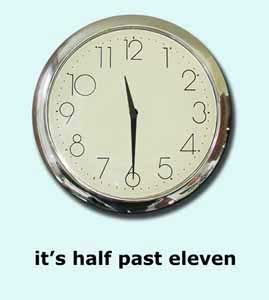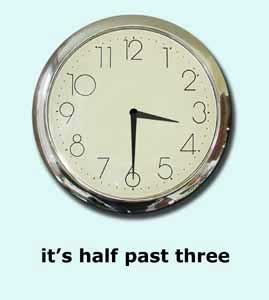#### example 4

When the minute hand is pointing to 9, we say that the time is "a quarter till/to _____."
The hours hand will be pointed in between two numbers. The hour of the day is still the lower number, but it is getting close to the next, higher number, so use the higher number in the "a quarter till/to _____" sentence.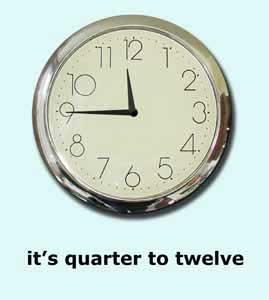### When minute hand is NOT pointing to 12, 3, 6 or 9

#### example 1

If the minute hand is between 1-29:
The small hand indicates the hour, and the big hand indicates the minute.
The hours hand will be pointed in between two numbers. The hour of the day is the lower number. In your mind, extend the minute hand all the way to the edge, and read how many minutes it has passed. Read the time as "it is ____ minutes after/past _____."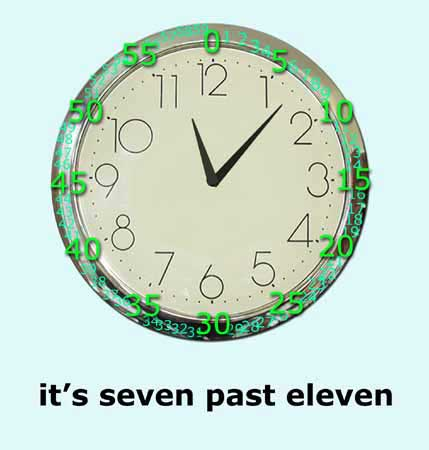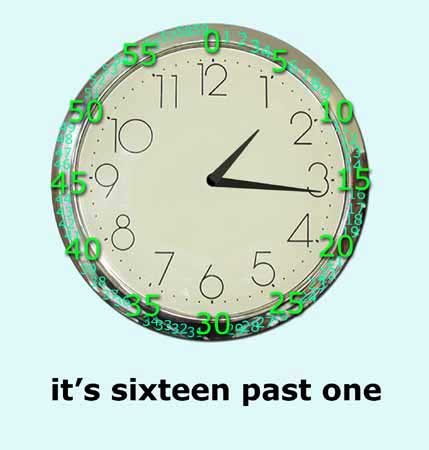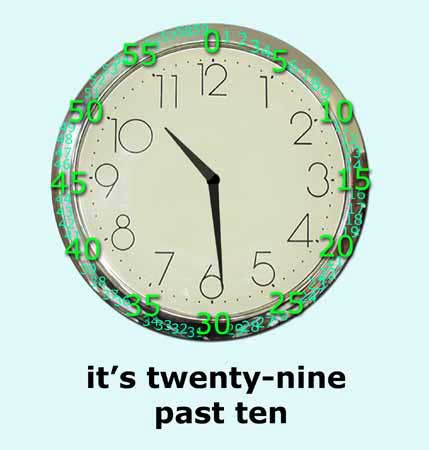#### example 2

If the minute hand is between 31-59:
The small hand indicates the hour, and the big hand indicates the minute.
The hours hand will be pointed in between two numbers. The hour of the day is still the lower number, but it is getting close to the next, higher number, so use the higher number.
In your mind, extend the minute hand all the way to the edge, and read how many minutes it has passed and then subtract the minutes from 60.
For example: 60-58=2, 60-50=10, 60-41=19.
It will tell you how many minutes are left till the next hour. Read the time as "it is ____ minutes till/to _____."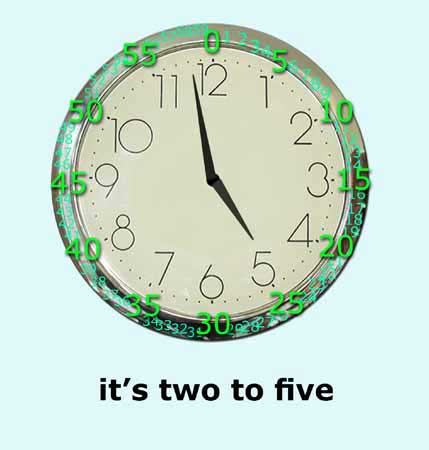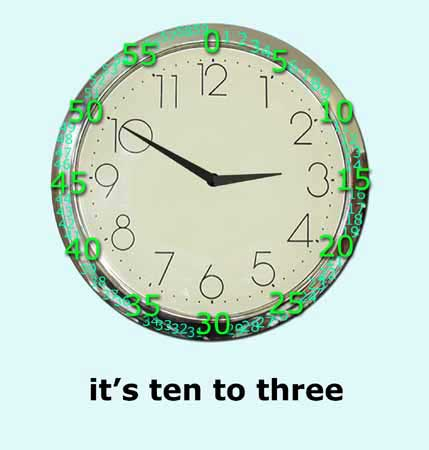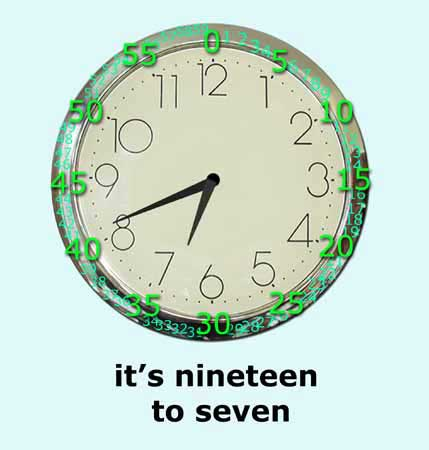Time is calculated in a variety of ways. For instance, time can be calculated in seconds, minutes, hours, days, weeks, and etc. Below are the different ways time is measured:

NameConversion
Second-
Minute60 seconds
Hour60 minutes
Day24 hours
Week7 days
Fortnight2 weeks
Month number of days in a month:
28, 29, 30, or 31
Quarter3 months
Year12 months
365 or 366 Days
52 weeks
Leap Year366 days